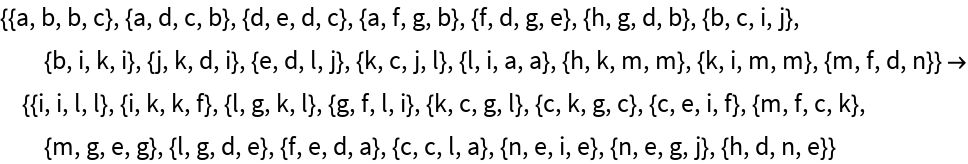#Function Repository Resource:

# CanonicalWolframModelRule

Attempt to find a canonical representation for a Wolfram model

Contributed by: Ed Pegg
 ResourceFunction["CanonicalWolframModelRule"][rule] attempts to find a canonical version of the specified Wolfram model rule. ResourceFunction["CanonicalWolframModelRule"][rule,"Letter"] produces a canonical letter form of the specified Wolfram model rule.

## Details and Options

The canonicalization follows several rules:
 1 Tuples are sorted by length, with longest tuples occurring first 2 Tuples of the same length on the same side of a rule are considered a rule part 3 A rule part with 3 tuples of length 2 has signature 32 4 Rule parts are ordered so that new alphabet terms are introduced with maximal frugality
ResourceFunction["CanonicalWolframModelRule"] uses a heuristic method and may not give a complete canonical form in all cases.

## Examples

### Basic Examples

Rename nodes and re-sort rules to find a canonical form:

 In:=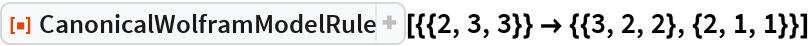Out=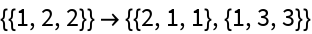In:=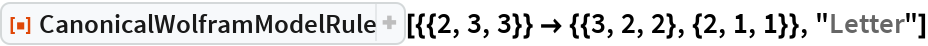Out=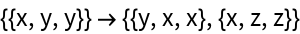In:=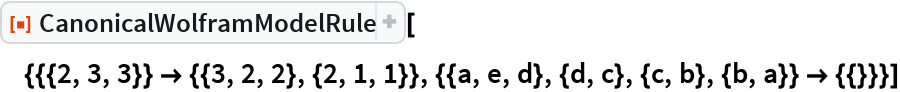Out=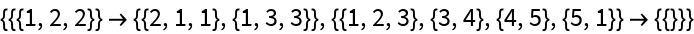In:=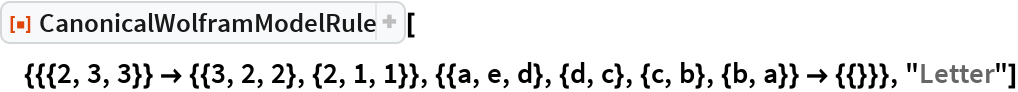Out=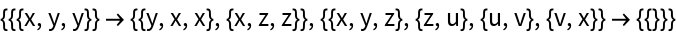A slightly more complicated case:

 In:=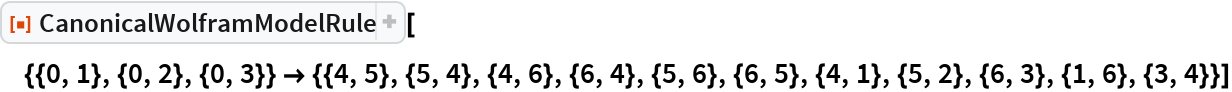Out=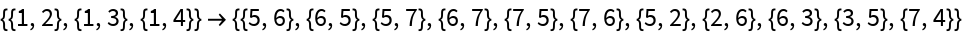In:=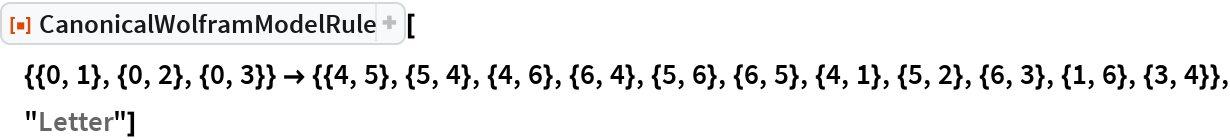Out=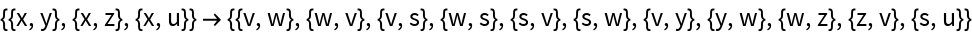CanonicalWolframModelRule can handle any names for elements:

 In:=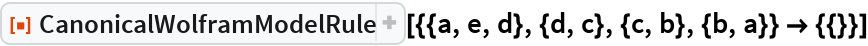Out=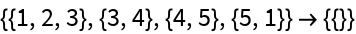In:=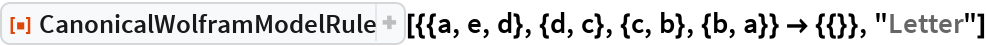Out=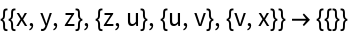Some difficult cases: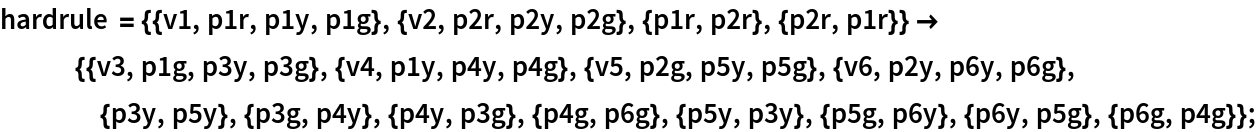In:=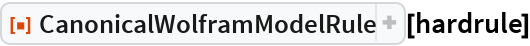Out=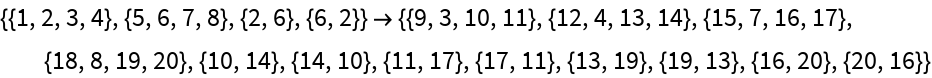In:=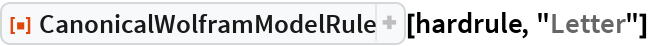Out=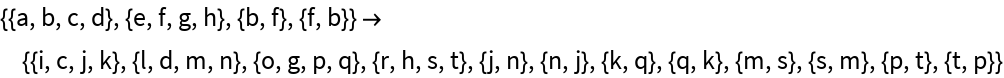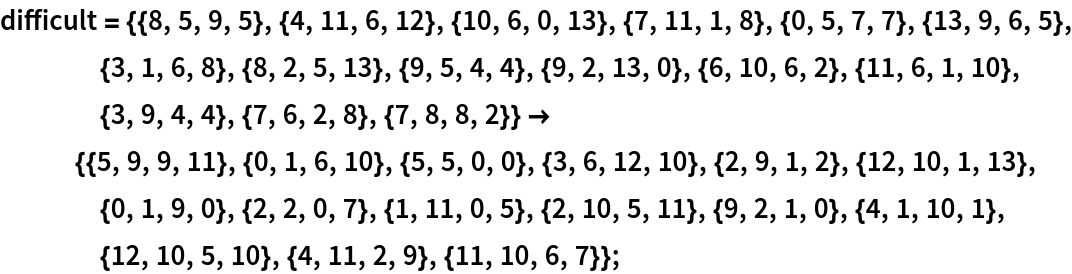In:=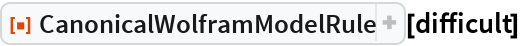Out=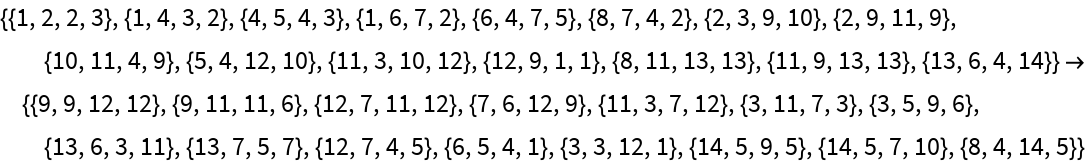In:=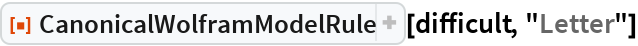Out=